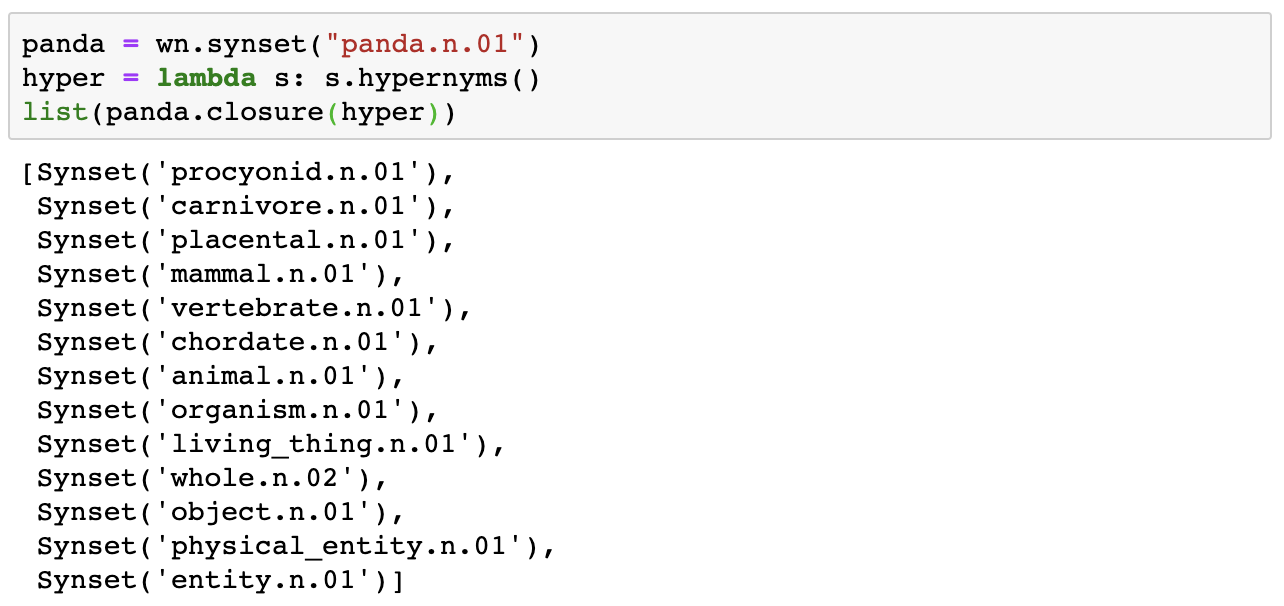# 1 Introduction of Natural Language Processing

Natural Language is a discrete/symbolic/categorical system.

There are many different levels in NLP. For instance:

Easy:

• Spell Checking

• Keywords Search

• Finding Synonyms

• Synonym: 同义词 noun

Medium

• Parsing information from websites, documents, etc.

• Parse: 对句子进行语法分析 verb

Hard

• Machine Translation

• Semantic Analysis (What is the meaning of query statement?)

• Coreference (e.g. What does “he” or “it” refer to given a document?)

Coreference: 共指关系，指代的词

## 1.2 How to represent words?

### 1.2.1 WordNet

• Use e.g. WordNet, a thesaurus containing lists of synonym sets and hypernyms (“is a” relationships).

hypernym: 上位词Problems with resources like WordNet

• Great as a resource but missing nuance (e.g. “proficient” is listed as a synonym for “good”, This is only correct in some contexts.)
• Missing new meanings of words
• Subjective
• Requires human labor to create and adapt
• Can’t compute accurate word similarity

### 1.2.2 Representing words as discrete symbols

In traditional NLP, we regard words as discrete symbols: hotel, conference, motel - a localist representation.

Words can be represented by one-hot vectors, one-hot means one 1 and the rest are 0. Vector dimension = number of words in vocabulary.

• Problem with words as discrete symbols:

For example, if user searches for “Seattle motel”, we would like to match documents containing “Seattle hotel”.

But:

$$hotel = [0\quad 0\quad 0\quad 0\quad 0\quad 1\quad \cdots \quad 0]\motel = [0\quad 0\quad 0\quad 0\quad 1\quad 0\quad \cdots \quad 0]$$

These two vectors are orthogonal. But for one-hot vectors didn’t shown there’s natural notion of similarity.

• Solution:

• Could try to rely on WordNet’s list of synonyms to get similarity?

• But it is well-known to fail badly: incompleteness, etc.
• Instead: Learn to encode similarity in the vectors themselves

### 1.2.3 Representing words by their context

• Distributional semantics: A word’s meaning is given by the words that frequently appear close-by.

• “You shall know a word by the company it keeps”(J.R. Firth 1957: 11)
• One of the most successful ideas of modern statistical NLP
• When a word “$w$” appears in a text, its context is the set of words that appear nearby(within a fixed-size windows).

• Use the many contexts of “$w$” to build up a representation of “$w$”.# 2 Word2Vec

• Word Vectors: In word2vec, a distributed representation of a word is used. Each word is represented by a distribution of weights across those elements. So instead of a one-to-one mapping between an element in the vector and a word, the representation of a word is spread across all of the elements in the vector, and each element in the vector contributes to the definition of many words.

Note: Word vectors are sometimes called word embeddings or word representations. They are a distributed representation.

## 2.1 Word2Vec: Overview

Word2vec (Mikolov et al. 2013) is a framework for learning word vectors.

Idea:

• We have a large corpus of text
• Every word in a fixed vocabulary is represented by a vector
• Go through each position $t$ in the text, which has a center word $c$ and context (“outside”) words $o$
• Use the similarity of the word vectors for $c$ and $o$ to calculate the probability of $o$ given $c$ (or vice versa)
• Keep adjusting the word vectors to maximize this probability

## 2.2 Word2Vec: Objective Function

Example windows and process for computing:

$$P(w_{t+j}|w_t)$$For each position, $t=1, \dots , T$ , predict context words within a window of fixed size $m$, given center word $w_j$.

$$Likelihood=L(\theta)=\prod_{t=1}^T\prod_{-m\leq j \leq m}P(w_{t+j}|w_t;\theta)$$

where $\theta$ is all variables to be optimized.

• The objective function $J(\theta)$ is the average negative log likelihood:

Sometimes, objective function called $cost$ or $loss$ function.

$$J(\theta)=-\frac{1}{T}\log L(\theta)=-\frac{1}{T}\sum_{t=1}^{T}\sum_{-m\leq j \leq m}\log P(w_{t+j}|w_t;\theta)$$

So, our target that maximizing predictive accuracy is equivalent to minimizing objective function.

Minimizing objective function $\Leftrightarrow$ Maximizing predictive accuracy.

How to calculate $P(w_{t+j}|w_t;\theta)$ ?

To calculate $P(w_{t+j}|w_t;\theta)$ use two vectors per word $w$:

• $v_w$ when $w$ is a center word
• $u_w$ when $w$ is a context word

Then for a center word $c$ and a context word $o$:

$$P(o|c)=\frac{\exp(u_o^Tv_c)}{\sum_{w\in V}\exp(u_w^Tv_c)}$$

In the formula above $Exponentiation$ makes anything positive, $u_o^Tv_c$ is dot product that compares similarity of $o$ and $c$. Large dot product = Large probability. Denominator $\sum_{w\in V}\exp(u_w^Tv_c)$normalize over entire vocabulary to give probability distribution.

The follow is an example of $softmax function$ $\mathbb{R}\rightarrow\mathbb{R}$

$$softmax(x_i)=\frac{\exp(x_i)}{\sum_{j=1}^{n}\exp(x_j)}=p_i$$

Softmax Function apply the standard exponential function to each element $x_i$ of the input vector $x$ and normalize these values by dividing by the sum of all these exponentials; this normalization ensures that the sum of the components of the output vector $p$ is 1.

The softmax function above maps arbitrary values $x_i$ to a probability distribution $p_i$

• $max$ because it amplifies probability of largest $x_i$
• $soft$ because it still assigns some probability to smaller $x_i$
• Frequently used in Deep Learning.

$\theta$ represents all model parameters. For example we have $V$ words and each vector of word has $d$ dimensional. And every word such as word $w$ have two vectors, one is the center word vector $v_w$ and another is the context vector $u_w$. So, $\theta \in \mathbb{R}^{2dV}$ , we can optimize these parameters by walking down the gradient.

$$\frac{\partial x^Ta}{\partial x}=\frac{\partial a^Tx}{\partial x}=a$$

Write out with indices can proof it.

We need to minimize

$$J(\theta)=-\frac{1}{T}\log L(\theta)=-\frac{1}{T}\sum_{t=1}^{T}\sum_{-m\leq j \leq m, j\neq0}\log P(w_{t+j}|w_t;\theta)$$

minimize $J(\theta)$ is equivalent to minimize $\log P(o|c)=\log \frac{\exp(u_o^Tv_c)}{\sum_{w=1}^V\exp(u_w^Tv_c)}$.

Take the partial of $\log P(o|c)=\log \frac{\exp(u_o^Tv_c)}{\sum_{w=1}^V\exp(u_w^Tv_c)}$, we can get the follow results:

$$\log P(o|c)=\log \frac{\exp(u_o^Tv_c)}{\sum_{w=1}^V\exp(u_w^Tv_c)}=\log \exp(u_o^Tv_c)-\log \sum_{w=1}^V\exp(u_w^Tv_c)$$

$$\frac{\partial P(o|c)}{\partial v_c}=u_o-\frac{\sum_{x=1}^V u_x\exp(u_w^Tv_c)}{\sum_{w=1}^V\exp(u_w^Tv_c)}=u_o-\sum_{x=1}^Vu_xP(x|c)$$

The statement above illustrate the skip-gram language model and how to update the parameters of $J(\theta)$.